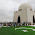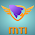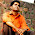# Swinburne’s Test for DC Machines

In this technique, the DC Generator or DC Motor is run as a motor at no load; with that losses of the DC machines are determined. When the losses of DC machine well-known, then we can find the efficiency of a DC machine in advance at any desired load. In DC machines this test is applicable only throughout the flux is constant at all load (DC Shunt machine and DC Compound Machine). This test maintains of two steps;

### Determination of Hot Resistance of Windings:

The resistance of armature windings and shunt field windings are measured with the help of a battery, ammeter and voltmeter. Since these armature and shunt filed resistances are measured while the DC machine is cold, it should be transformed to values equivalent to the temperature at which the DC machine would work at full load. These values are measured generally when the room temperature increases above 40oC. Take on the hot resistance of armature winding and shunt field winding be Ra and Rsh correspondingly.

### Determination of Constant Losses:

On no load the DC machine run as a motor with the supply voltage is varied to the normal rated voltage. With the use of the field regulator R the motor speed is varied to run the rated speed which is shown in the figure.
Let
V = Supply Voltage
Ish = Shunt Field current ready by A2
No load armature current Iao = I0 – Ish
No load Input power to motor = VI0
No load Input power to motor = VIa0
= V (I0 – Ish)

As the output power is nil, the no loads input power to the armature provides Iron loss, armature copper loss, friction loss and windage loss.

Constant loss Wc = Input power to Motor – Armature copper loss
Wc = VI0 – (I0 – Ish2Ra)

As the constant losses are identified, the efficiency of the DC machine at any loads can be determined. Suppose it is desired to determine the DC machine efficiency at no load current. Then,
Armature current Ia = I-Ish (For Motoring)
Ia = I+Ish (For Generating)

#### To find the Efficiency when running as a motor:

Input power to motor = VI
Armature copper loss =Ia2Ra = (I-Ish2Ra)
Constant Loss = Wc
Total Loss = (I-Ish2Ra)+Wc
Motor Efficiency η = (Input power – Losses)/ Input
η = {VI – (I-Ish2Ra)} / VI

#### To find the Efficiency when running as a Generator:

Output Power of Generator = VI
Armature copper loss =Ia2Ra = (I+Ish2Ra)
Constant Loss = Wc
Total Loss = (I+Ish2Ra)+Wc
Motor Efficiency η= Output power/ (Output power + Losses)
η  = VI / {VI + (I+Ish2Ra) + Wc}

#### Merits:

• Since this test is no load test, power required is less. Hence the cost is economic.
• The efficiency of the machine can be found very easily, because the constant losses are well known.
• This test is appropriate.

#### Demerits:

• When the DC machine is loaded, this test does not deliberate the stray load loss that occurs.
• Using this method we cannot check the DC machine performances at full load.
Example: It does not indicate the commutation performance is satisfactory on full load and cannot indicate the specified limit of temperature rise.
• Using this test we cannot determine the accurate efficiency of DC machine, because iron loss at actual loads is greater than at no load. This is primarily owed to armature reaction interfere with field.5 CommentsComments

#### Read Comment Policy We have Zero Tolerance to Spam. Chessy Comments and Comments with Links will be deleted immediately upon our review.

1.Hi, Please Send me Template , Thanks
[Superbests9835@gmail.com]

1.You need to pay \$69. If interested let me know. Then i will send the link from where to purchase.

2.3.never you will get

4.Hi....
First I sorry, I am doing comment not on behalf of this post...
I am gonna to buy this template, before buying this In would like to know experience on the same.. specially on SEO part...